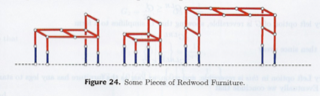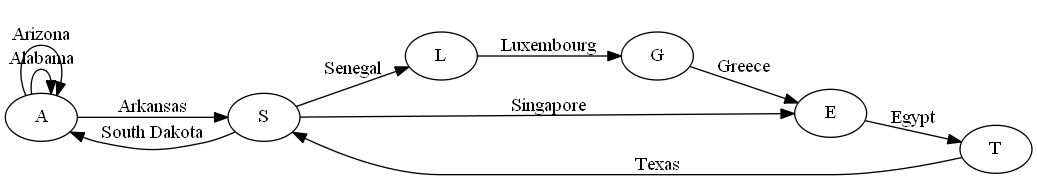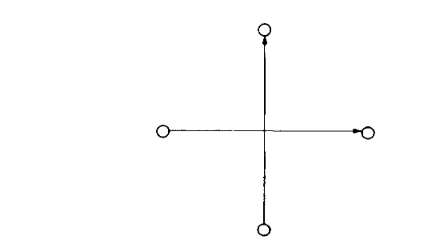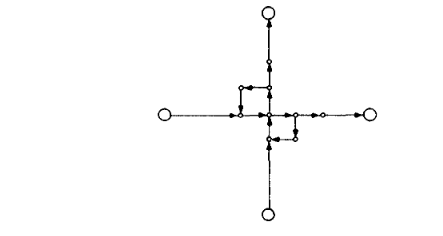# Tag Archives: Difficulty 5

## Crossword Puzzle Construction

Closing in on the end of the section, this is a “private communication” problem that I think I figured out myself.

The problem: Crossword Puzzle Construction.  This is problem GP14 in the appendix.

The description: Given a set W of words (strings over some finite alphabet Σ), and an n x n matrix A, where each element is 0 (or “clear”) or 1 (or “filled”).  Can we fill the 0’s of A with the words in W?  Words can be horizontal or vertical, and can cross just like crossword puzzles do, but each maximally contiguous horizontal or vertical segment of the puzzle has to form a word.

Example: Here’s a small grid.  *’s are 1, dashes are 0:

 * – – – * – – – – – – – – – – * * – – * * * * – *

Suppose our words were: {SEW, BEGIN, EAGLE, S, OLD, SEA, EGGO, WILLS, BE, SEA, NED}.  (Notice the lone “S” is a word.  That’s different from what you’d see in a normal crossword puzzle)

We can fill the puzzle as follows:

 * S E W * B E G I N E A G L E * * O L D * * * S *

Notice that we can’t use the first two letters of “BEGIN” as our “BE”, because the word continues along.  That’s what the “maximally contiguous” part of the definition is saying.

Reduction: From X3C. We’re given a set X with 3q elements, and a collection C of 3-element subsets of X.  We’re going to build a 3q x q puzzle with no black squares. (We’ll get back to making this a square in a minute)  Each word in W will be a bitvectorof length 3q, with a 0 in each position that does not have an element, and a 1 in the positions that do.  So, if X was {1,2,3,4,5,6,7,8,9} the set {1,3,5} would be 101010000

We also add to A the 3q bitvectors that have exactly one 1 (and 0’s everywhere else). The goal is to find a subset of C across the “rows” of the puzzle, such that the “columns” of the puzzle form one of the bitvectors.  If we can form each of the bitvectors, we have found a solution to X3C.  If we have a solution to X3C, we can use the elements in C’ and place them in the rows of the 3q x q puzzle block to come up with a legal crossword puzzle.

We’re left with 2 additional problems:  The grid needs to be a square, instead of a 3q x q rectangle, and the legal crossword puzzle solution needs to use all of the words in W, not the the ones that give us a C’.  We can solve both by padding the grid with blank squares.  Spaced out through the blank spaces are 1 x 3q sections of empty space surrounded by black squares.  We can put any word in C-C’ in any of these sections, and that’s where we’ll put the words that are not used.

(This also means we’ll have to add 3x|(C’-C)| 1’s and (3q-3)|(C’-C)| 0’s to our word list for all of the 1-length words in those  columns.)  Then we add enough blank spaces around the grid to make it a square.

Difficulty: 5 if I’m right, mainly because of the extra work you have to do at the end.  The comments in G&J say that the problem is NP-Complete “even if all entries in A are 0”, which is usually a hint that the “actual” reduction used an empty square grid.  I wonder if that reduction doesn’t have my hacky stuff at the end.

## Left-Right Hackenbush for Redwood Furniture

Here’s an interesting problem, but a hard one to explain.  Most of what I’m doing here comes from the very cool “Winning Ways for Your Mathematical Plays” book by Berlekamp, Conway, and Guy, which I hope at some point in the future to have the time to really dig deeply into.  But for now, I’ll just use it as a reference to this week’s problem.

The problem: Left-Right Hackenbush for Redwood Furniture.  This is problem GP12 in the appendix.

The description: Ok, here we go.  First, a Hackenbush problem consists of an undirected, connected graph.  The edges of the graph are marked as “Left” or “Right”(though the book has some very nice colored pictures, where the edges are labeled “Blue” and “Red”).  Some of the vertices are on the ground (In G&J’s definition, there is one ground vertex, but it’s equivalent to having several vertices that are all on the ground).

On Left’s turn, they remove a blue edge and then all edges that are not connected to the ground are removed.  On Right’s turn, they remove a red edge, and then all edges that are not connected to the ground are removed.  A player loses if there are no remaining edges of their color.

redwood furniture Hackenbush instance is one where:

• No red edges touch the ground
• Each blue edge (or “foot”) has one end on the ground and the other touches a unique red edge (the “leg”)

Here are some redwood furniture instances from the book:The “value” of a Hackenbush position is the number of “spare” moves (with optimal play) one player has after the other player loses.  A value of 0 means that whoever’s turn it is will lose (on an empty board).  The definition can be extended to fractional values.  For example, a value of 1/2 for (say) Left means that if we made two copies of the game, we would end up with a situation with a value of 1 for Left.

So, the question is, for some Redwood Furniture graph, and some K, is the value <= 2-K?

Reduction:

I’m just going to sketch the process here since it takes several pages of the book (and depends on results and ideas from earlier in the book).

They show:

• The value of any redwood furniture graph is 2-N for some N.  In the degenerate case, the graph with just one Left edge has a value of 1. (=20)
• On Left’s turn, they will always remove a foot (by definition, that’s all they have).  On Right’s turn, they should make a move that does not disconnect the graph, if possible.
• A “Bed” is a redwood furniture graph where every red edge that is not a leg connects to a leg.  It has value 2-(m+1), where m is the largest number of moves that do not disconnect the bed.
• The value of the game depends on how many extra moves Red has to get down to just a bed.
• To find m, you need to know the size of the largest redwood tree (a tree is a graph that will be disconnected by the removal of any edge) that contains all of the legs.
• The edges of the bed (the red edges that are not legs) form a bipartite graph.  So finding m is equivalent to the set covering problem, where the elements of the set are the vertices, and the edges are the sets.

Here’s how I think the Set Covering reduction works.  Given a set covering instance: a set S of elements, and a collection C of subsets of S, we’ll build a bipartite graph.  One set of the bipartite graph will correspond to the elements of S (and will be on the legs of the furniture graph).  The other set will correspond to the elements in C (and will be the vertices in the bed that are not in the legs).  An edge will go from each “C vertex” to each “S vertex” in its set.  Now, the cover is the set of vertices from the bed that cover all of the legs.

The book says you want the “smallest redwood tree which contains all of the legs”, which I think is the same thing (smallest number of extra vertices), but I’m not 100% confident since the Hackenbush game involves removing edges, and we’re choosing vertices in the cover.

I’m a little sad that the book does such a great job describing the game, and the value function, and then glosses over the reduction part (and uses misleading terms like “Minimum Spanning Tree of a Bipartite Graph”, which is a polynomial problem).  The actual reduction in G&J is to a private communication, so that’s not much help.

Difficulty: Boy, I don’t know, I think it depends on where you start from.  If my Set Cover reduction is the right one, and all you ask a student to do is that, it’s probably a 4.  If you’re going to make them prove all of the things I glossed over about the value number, then it probably goes up to at least an 8.

## Planar Geography

In the process of reading up on the next problem in the appendix, I saw this nice reduction for a generalization of Geography that we used last week and will use next week.  So I thought it would make a nice addition to post it here.

The problem: Planar Geography.  This problem is not in the appendix (though the reference to this problem and the paper with this reduction appears in the notes to the “Generalized Geography” problem).

The description: Given an instance of the Generalized Geography problem, but where the graph G is planar, does Player 1 have a forced win?

Example: The example we had in the Generalized Geography problem is actually a planar graph:Reduction: The paper by Lichtenstein and Sipser which has the reduction for next week’s NxN Go problem has this in the ramp-up.

What we’re doing to do is re-build the graph that was created in the Generalized Geography reduction.  That graph may not be planar. Any time the graph has two edges that cross:We replace it with the following construction(figures are on pages 395 and 396 of the paper).

The important things to realize are these:

1) If we use this on the graph generated from the original Geography Construction, it will never be the case that we will use both crossing edges in one solution.  This is an important point that isn’t really addressed in the paper.  The Lichtenstein and Sipser paper has a slightly different reduction for Generalized Geography where it’s easier to see.

2) Suppose it’s player 1’s turn, and they enter the intersection going up.  Then it will be their turn again once they hit the middle point.  The point of the extra loop structures (instead of just adding a vertex at the intersection point) is to create a situation where if player 1 decides to turn right, player 2 can turn down, and make player 1 lose.  This works in both directions.

Difficulty: I was hoping that this would be a good difficulty 4 or so problem in the middle of all of these really hard ones, but the whole “you’ll never use this intersection twice” problem makes the construction hard to see, and it’s a tough thing to think about why it matters, even if you told the student the rule.  Maybe this is a 5 if you give them that information.